Chemical and Process Engineering Resources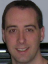Basics of Industrial Heat Transfer

Nov 08 2010 01:30 PM | Chris Haslego in Heat Transfer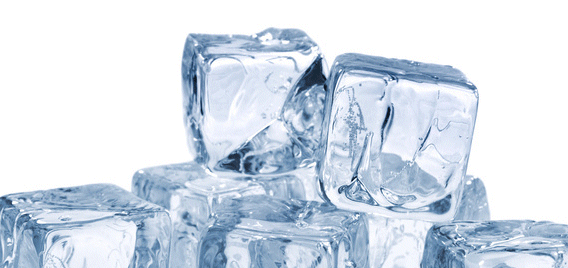Examining the "Heat Exchanger Equation"

If we take a closer look at the heat exchanger equation, it's worth noting some assumptions that are made in its derivation. First, the overall heat transfer coefficient and the specific heat (also called heat capacity) of the fluids are assumed to remain constant through the heat exchanger.
If we look at the change in the heat capacity of water, for example, over a reasonable temperature range, here is what we find:

Specific heat of water at 100 Â°F and atmospheric pressure = 0.9979 Btu / lb Â°F
Specific heat of water at 210 Â°F and atmospheric pressure = 1.0066 Btu / lb Â°F
So, we can see that this is a fairly reasonable assumption for water and it remains reasonable for most industrial fluids. The specific heat of a substance is defined as the amount of heat required to raise the temperature of one pound of the substance by a single degree Fahrenheit (other units can apply as well).
The overall heat transfer coefficient is a calculated variable based on the physical properties of the fluids involved in the heat transfer (hot and cold) as well as the geometry and type of heat exchanger to be used. We'll examine this closer a little later.
The log mean temperature difference or LMTD is used to describe the average temperature difference throughout the exchanger. The difference between the temperatures of the fluids provides the "driving force" for the heat transfer to occur. The larger the temperature difference, the smaller the required heat exchanger and vice versa.
You'll notice from our T-Q diagram used to explain the equations: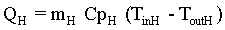that it appears that the temperature difference between the fluids remains almost constant throughout the heat exchanger. This is rarely the case. Let's look at a more practical example. Let's assume that a process stream containing water at 200 Â°F is to be cooled to 150 Â°F using cooling tower water available at 85 Â°F. It is common practice in industry to return cooling tower no higher than 120 Â°F. In other words, the cooling tower water flow must be such that its outlet temperature from the heat exchanger is less than 120 Â°F. The reason for this is that cooling tower water often contains treatment chemicals that can plate out onto heat transfer surfaces and cause severe fouling or degradation of the heat transfer rate at elevated temperatures.
Here is what the T-Q diagram may look like for our example case: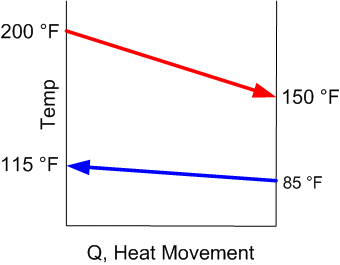Figure 3: T-Q Diagram for the Example Problem

You can see that the temperature difference between the two streams will vary widely. This is why the log mean temperature difference is used.Here is how the log mean temperature difference works: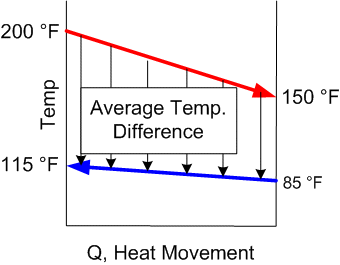Figure 4: Graphical Representation of the Log Mean Temperature Difference

So, for a heat exchanger as described above, we calculate the LMTD as follows: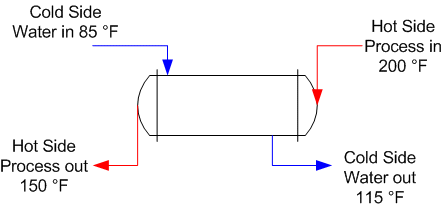Figure 5: Exchange Heat Exchanger DutyEq. (4)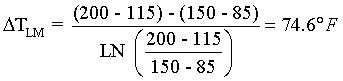There can be special cases where the LMTD equation shown above is not applicable. Consider the case below:Figure 6: Special Case for Calculating LMTD

If you tried apply the LMTD equation to this special case, you'd find that the result would be zero. In this case the LMTD is the same as the temperature difference on each "end" of the heat exchanger, or 100 Â°F.

A Brief Word on Flow Direction

Notice that up to this point, the two fluids considered in a heat exchanger have been moving in opposite directions to one another. This is known as counter-current flow. This is the predominantly preferred flow direction because it results in higher temperature difference driving forces within the heat exchanger, thus minimizing the heat transfer area required.

The other flow configuration, where the fluids flow in the same direction, is called co-current flow. Co-current flow, while it is rarely used, does have the advantage of lowering the heat exchanger wall temperature on the hot side fluid. This can be useful for temperature sensitive fluids or as a means of minimizing deposits that are temperature sensitive.

The Temperature Correction Factor, f

The temperature correction factor, f, is used to correct the log mean temperature difference for heat exchangers than lack truly counter-current flow. Many different heat transfer technologies lack truly counter-current flow patterns as a result of their inherent mechanical design. Generally, the value for f should be between 0.75 of 0.97. There are cases when this value can be taken as one, but only if the flow in the exchanger is purely counter-current. There are countless charts available to look up the temperature correction factor for a given configuration.

The Overall Heat Transfer Coefficient

The overall heat transfer coefficient describes the rate of heat transfer in the heat exchanger. Generically, it is described by the following equation: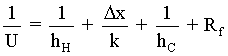Eq. (5)

Where:
U = overall heat transfer coefficient (Btu / h ft2 Â°F)
hH = hot side heat transfer coefficient
hC = cold side heat transfer coefficient
Delta x = exchanger wall thickness
k = exchanger wall material thermal conductivity
Rf = fouling coefficient (h ft2 Â°F / Btu)
The equation for the overall heat transfer coefficient is often reduced to the following: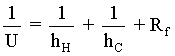Eq. (6)

Because the term Delta x / k seldom has any significant impact on the overall U-value.
The overall heat transfer coefficient can either be calculated, looked up in reference materials for a given duty, estimated from past plant experience, or supplied by a heat exchanger vendor.

Heat Transfer Articleschengineer

Heat Exchanger iOS application

https://itunes.apple...633422031?mt=8#!

I think it may be helpful to introduce an application (Heat Exchanger) which can be used in solving heat transfer equation and designing double pipe heat exchanger with following features:

1. Calculating each variable in energy balance equation
2. Designing double pipe heat exchanger with real size of pipe
3. Calculating overall heat transfer coefficient(U)
4. User can define any fluid with specific properties or select from most common fluids used in industrial application
5. Data can be edited in both SI and USCS unit standards.
6. User friendly UI designdebu

THANKS FOR THE ARTICLE. IF YOU SO KIND TO GIVE THE INFORMATION ABOUT DIRECT CONTACT JUICE HEATERS CALCULATION. THE HEATERS MAINLY USED IN SUGAR INDUSTRIES TO TEMPERATURE THE JUICE.monak

Thanks

•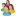Industrial Forum

•Student Forum

•Refining Forum

•Simulation Forum

•Relief Devices Forum

•Tank Venting Forum

•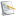Separation Technology

•Calculations and Tips

•Fluid Flow

•Heat Transfer

•Maintenance and Repair

•Utilities

•Safety and Pressure Relief

•Bulk Solids

•Process and Reactions

•Energy

•Other Topics

•For Students

•Physical Properties

•Archived Articles